# The n Variables, n Equations Rule

We have just seen that two linear equations of two variables will always have a single solution where the two lines that they represent cross in the coordinate plane. That’s an example of an important rule called the n Variables, n Equations Rule. In the case of two variables, two distinct lines will give us a solution. In general, to solve for n variables, you need n distinct equations.

## Examples of n Variables, n Equations

In this case, we have one variable and one equation, so the situation is solvable (we simply subtract five from both sides):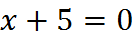This equation, however, is not completely solved: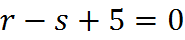We have two variables and only one equation. To solve in this case, we will need another equation that includes r and/or s, such as r + s = 1.

Here is a situation with three variables and three equations: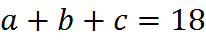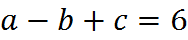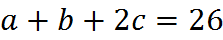Here, we have sufficient information to solve. We can solve by substitution or combination, as discussed in the last section.

## Distinct Equations

When you are looking for n equations, you must ask yourself whether the equations at hand are distinct. Let’s look at a modified version of the previous example: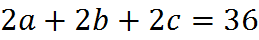This might look like three equations and three variables, at first. However, the first and the second equation are not truly distinct, because the second equation is simply twice the first one. So, in this situation, we are missing an equation in order to be able to solve.

We can give a reason for why this is true, using a two-dimensional example. The equation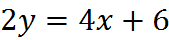is just twice the equation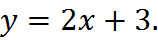And, in fact, they are graphs of the same line. We can see this by finding points on both lines and finding the slopes; each and every point that fits one equation will fit the other. The equations are completely equivalent, and the lines lie exactly on top of each other. That gives a visualization in the coordinate plane of how two equations might differ trivially in algebraic appearance but actually be the same.

## Applications of the n Variables, n Equations Rule

Here are the primary applications of the n variables, n equations rule:

· On Problem Solving questions, we can use the rule to stay organized. For example, on a word problem, we often have to translate words into algebra and then solve. When we know the question has an answer – because we have to find it! – and there are two unknowns, we know that we are probably looking for two linear equations.
<![if !supportLineBreakNewLine]>

· Similarly, we can use the rule to check our work. If we solved a situation that had three variables and there were only two equations, we might have done something wrong (but see the next section on this point).
<![if !supportLineBreakNewLine]>

· You will probably use the rule at least once when you sit for the exam to answer Data Sufficiency questions. The n variables, n equations rule is well suited for Data Sufficiency, because the rule answers the question, “Do we have enough information to solve?” and that’s the subject of every single Data Sufficiency question.

## Fortuitous Algebra

As we just saw, in some cases there appear to be n equations, but the equations are not distinct, and so we have less information than we might of thought and not enough to solve for all the variables. There is an opposite kind of special case when it comes to n variables and n equations. It doesn’t have a proper mathematical name, so in the GMAT Free course we refer to it simply as “fortuitous algebra.”

Consider this example, which is similar but different to the examples above: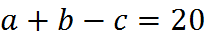In this case, we have a situation with two equations and three variables, so it looks like we are not going to be able to answer the question. However, even though we can’t solve for all the variables completely, maybe the question at hand isn’t asking us to. Suppose this equation asked us, “What is a + b?” In that case, we could add the two equations together (or solve for c and substitute) to obtain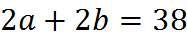Then, dividing both sides by 2, we have our answer: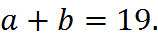The reason we could solve in this case was that the question didn’t ask us to solve completely. Notice that we never found out the exact values of a and b; we got only their sum. We couldn’t solve completely, but we solved for what was asked of us. You could say that we got lucky, and therefore we call this “fortuitous algebra.”

Fortuitous algebra appears on GMAT questions, so you must keep it in mind when using n variables, n equations.

## Practice Questions

Linear Equations with Fractions:
http://www.gmatfree.com/linear-equations-with-fractions

Counts and Prices:
http://www.gmatfree.com/counts-and-prices

Fortuitous Algebra:
http://www.gmatfree.com/fortuitous-algebra

Welcome! You are encouraged to register with the site and login (for free). When you register, you support the site and your question history is saved.